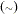The MCMC Procedure
 Specifying a New Distribution

To work with a new density that is not listed in the section Standard Distributions, you can use the GENERAL and DGENERAL functions. The letter “D” stands for discrete. The new distributions have to be specified on the logarithm scale.

Suppose that you want to use the inverse-beta distribution:The following statements in PROC MCMC define the density on its log scale:

```a = 3; b = 5;
const = lgamma(a + b) - lgamma(a) - lgamma(b);
lp = const + (a - 1) * log(alpha) - (a + b) * log(1 + alpha);
prior alpha ~ general(lp);
```

The symbol lp is the expression for the log of an inverse-beta (a = 3, b = 5). The function general(lp) assigns that distribution to alpha. Note that the constant term, const, can be omitted as the Markov simulation requires only the log of the density kernel.

When you use the GENERAL function in the MODEL statement, you do not need to specify the dependent variable on the left of the tilde. The log-likelihood function takes the dependent variable into account; hence there is no need to explicitly state the dependent variable in the MODEL statement. However, in the PRIOR statements, you need to explicitly state the parameter names and a tilde with the GENERAL and DGENERAL functions.

You can specify any distribution function by using the GENERAL and DGENERAL functions as long as they are programmable with SAS statements. When the function is used in the PRIOR statements, you must supply initial values. This can be done in either the PARMS statement (PARMS Statement) or within the BEGINCNST and ENDCNST statements (BEGINCNST/ENDCNST Statement).

It is important to remember that PROC MCMC does not verify that the GENERAL function you specify is a valid distribution—that is, an integrable density. You must use the function with caution.Previous Page | Next Page | Top of Page